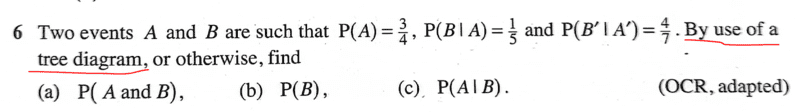# Solve the given problem that involves probability

Gold Member
Homework Statement:
See attached
Relevant Equations:
ProbabilityI would like to know how one can use the tree diagram...hence my post... otherwise, i was able to solve problem as follows,

a. ##P(A∩B)= \dfrac{3}{4} ×\dfrac{1}{5}=\dfrac{3}{20}##

b. ## P(B/A')=\dfrac{P(B)-\dfrac{3}{20}}{P(A')}##

##\dfrac{3}{7}=\dfrac{P(B)-\dfrac{3}{20}}{\dfrac{1}{4}}##

...

##P(B)=\dfrac{9}{35}##

c. ## P(A/B)=\dfrac{3}{20} ×\dfrac{35}{9}=\dfrac{7}{12}##

#### Attachments

Homework Helper
Gold Member
2022 Award
Homework Statement:: See attached
Relevant Equations:: Probability

View attachment 323090

I would like to know how one can use the tree diagram...hence my post... otherwise, i was able to solve problem as follows,

a. ##P(A∩B)= \dfrac{3}{4} ×\dfrac{1}{5}=\dfrac{3}{20}##

b. ## P(B/A')=\dfrac{P(B)-\dfrac{3}{20}}{P(A')}##

##\dfrac{3}{7}=\dfrac{P(B)-\dfrac{3}{20}}{\dfrac{1}{4}}##

...

##P(B)=\dfrac{9}{35}##

c. ## P(A/B)=\dfrac{3}{20} ×\dfrac{35}{9}=\dfrac{7}{12}##
See Conditional Probability Tree at https://www.cuemath.com/data/probability-tree-diagram/